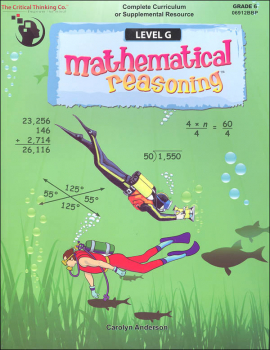# Mathematical Reasoning Level G (Gr. 6)

# BM-025350

Bargain Price: \$22.14
No more Bargain Versions available to purchase.Item #: BM-025350 9781601443144 6

#### Publisher Description:

Forget boring math lessons and dreaded drill sheets. This fun, colorful 448-page book uses engaging lessons with easy-to-follow explanations, examples, and charts to make sixth grade mathematical concepts easy to understand. It can be used as a textbook or a comprehensive workbook with your textbook to teach the math skills and concepts that students are expected to know in sixth grade—and several concepts normally taught in seventh grade. This book emphasizes problem-solving and computation to build for success in higher-level math and math assessments. Every lesson is followed with a variety of fun, colorful activities to ensure concept mastery. The lessons and activities spiral slowly, allowing students to become comfortable with concepts, but also challenging them to continue building their problem-solving skills. This book teaches more than mathematical concepts; it teaches mathematical reasoning, so students learn to devise different strategies to solve a wide variety of math problems. It is written to the standards of the National Council of Teachers of Mathematics.

CONTENTS

• Age
• Analyze Data
• Angles
• Area
• Bar Graph
• Calendar
• Capacity
• Characteristics
• Circle Graphs
• Circumference
• Coins
• Congruence
• Coordinates
• Count
• Data Analysis
• Data Collection
• Decimals
• Division
• Equations
• Equivalence
• Estimate
• Expanded Notation
• Fractions
• Graphs
• Inequalities
• Language
• Length
• Manipulative
• Match
• Mean
• Median
• Mode
• Money
• Multiplication
• Negative Numbers
• Number Lines
• Odd/Even
• Order
• Parallel
• Patterns
• Perimeter
• Perpendicular
• Place Value
• Prediction
• Prime/composite
• Probability
• Properties
• Proportion
• Ratio
• Real World Problems
• Reflection/Translation/Rotation
• Regrouping
• Rounding
• Rulers
• Shapes
• Subtraction
• Survey
• Symmetry
• Tables
• Temperature
• Time
• Variables
• Weight
• Whole Numbers
• Word Problems
• Primary Subject
Mathematics
6
ISBN
9781601443144
Format
Paperback Book
Brand Name
Critical Thinking Company
Weight
3.05 (lbs.)
Dimensions
11.0" x 8.5" x 1.25"